Wheels and Pulleys

Note: The below formulas are valid using metric SI units, with forces expressed in newtons, and lengths in meters or millimeters. (See note on page 174 concerning weight and mass.)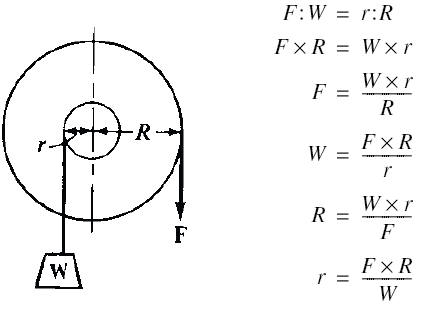The radius of a drum on which is wound the lifting rope of a windlass is 2 inches. What force will be exerted at the periphery of a gear of 24 inches diameter, mounted on the same shaft as the drum and transmitting power to it, if one ton (2000 pounds) is to be lifted? Here W = 2000; R = 12; r = 2.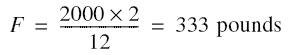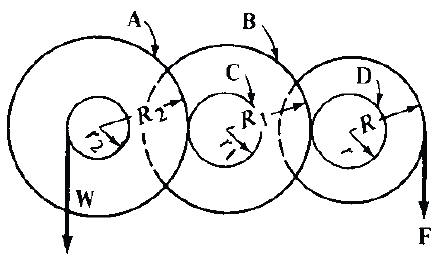A, B, C and D are the pitch circles of gears.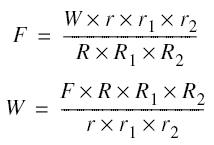Let the pitch diameters of gears A, B, C and D be 30, 28, 12 and 10 inches, respectively. Then R2 = 15; R1 = 14; r1 = 6; and r = 5. Let R = 12, and r2 = 4. Then the force F required to lift a weight W of 2000 pounds, friction being neglected, is: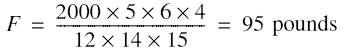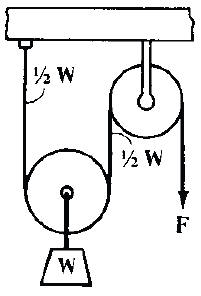F = 1⁄2WThe velocity with which weight W will be raised equals one-half the velocity of the force applied at F.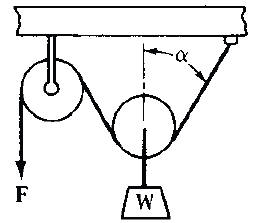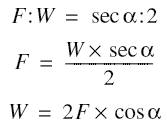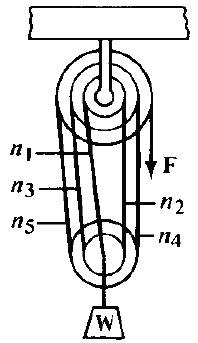n = number of strands or parts of rope (n1, n2, etc.).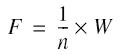The velocity with which W will be raised equals 1/n of the velocity of the force applied at F. In the illustration is shown a combination of a double and triple block. The pulleys each turn freely on a pin as axis, and are drawn with different diameters, to show the parts of the rope more clearly. There are 5 parts of rope. Therefore, if 200 pounds is to be lifted, the force F required at the end of the rope is: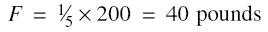Differential Pulley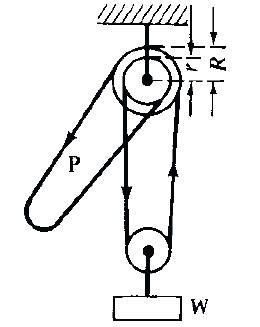In the differential pulley a chain must be used, engaging sprockets, so as to prevent the chain from slipping over the pulley faces.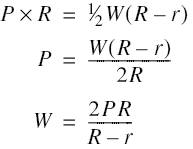The Chinese windlass is of the differential motion principle, in that the resultant motion is the difference between two original motions. The hoisting rope is arranged to unwind from one part of a drum or pulley onto another part differing somewhat in diameter. The distance that the load or hook moves for one revolution of the compound hoisting drum is equal to half the difference between the circumferences of the two drum sections.

Recherche personnalisée